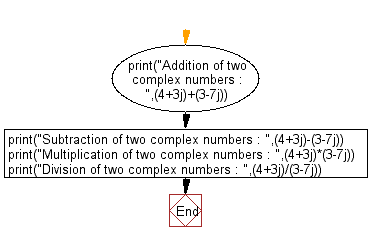﻿ Python Math: Add, subtract, multiply and division of two complex numbers - w3resource# Python Math: Add, subtract, multiply and division of two complex numbers

## Python Math: Exercise-33 with Solution

Write a Python program to add, subtract, multiply and division of two complex numbers.

Sample Solution:-

Python Code:

``````print("Addition of two complex numbers : ",(4+3j)+(3-7j))
print("Subtraction of two complex numbers : ",(4+3j)-(3-7j))
print("Multiplication of two complex numbers : ",(4+3j)*(3-7j))
print("Division of two complex numbers : ",(4+3j)/(3-7j))
```
```

Sample Output:

```Addition of two complex numbers :  (7-4j)
Subtraction of two complex numbers :  (1+10j)
Multiplication of two complex numbers :  (33-19j)
Division of two complex numbers :  (-0.15517241379310348+0.6379310344827587j)
```

Pictorial Presentation:Flowchart:## Visualize Python code execution:

The following tool visualize what the computer is doing step-by-step as it executes the said program:

Python Code Editor:

Have another way to solve this solution? Contribute your code (and comments) through Disqus.

What is the difficulty level of this exercise?

Test your Python skills with w3resource's quiz

﻿

## Python: Tips of the Day

Python: Get the Key Whose Value Is Maximal in a Dictionary

```>>> model_scores = {'model_a': 100, 'model_z': 198, 'model_t': 150}
>>> # workaround
>>> keys, values = list(model_scores.keys()), list(model_scores.values())
>>> keys[values.index(max(values))]
'model_z'
>>> # one-line
>>> max(model_scores, key=model_scores.get)
'model_z'
```